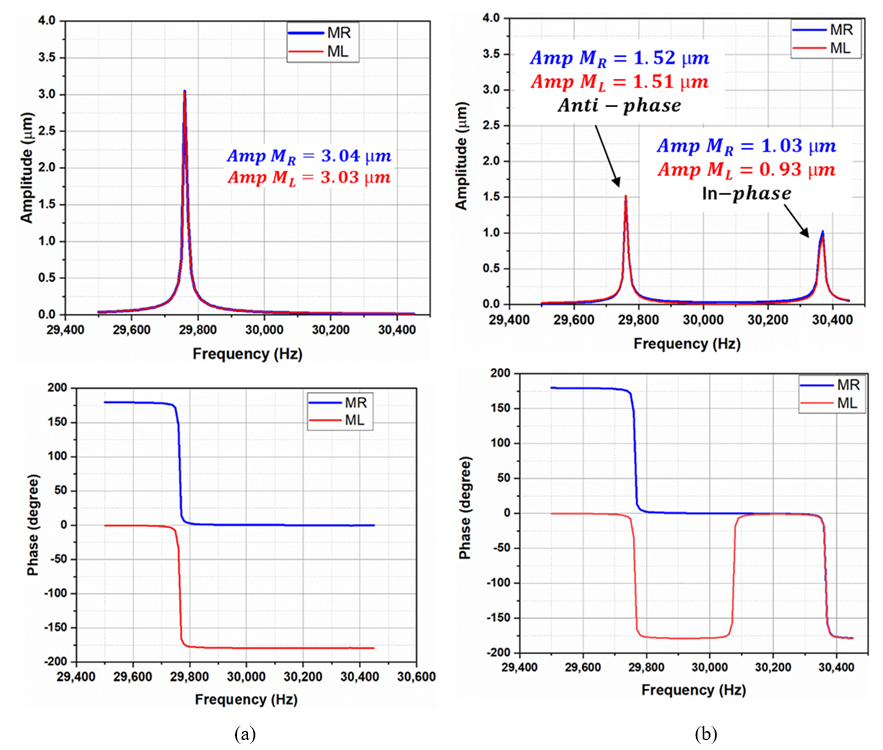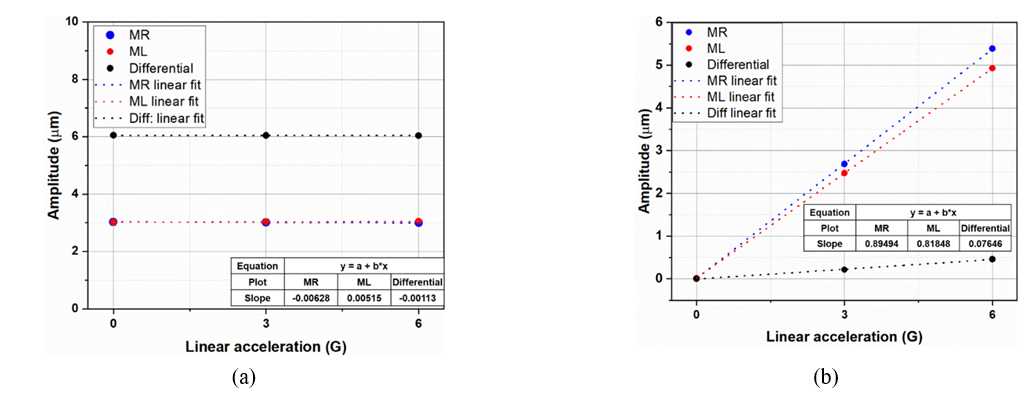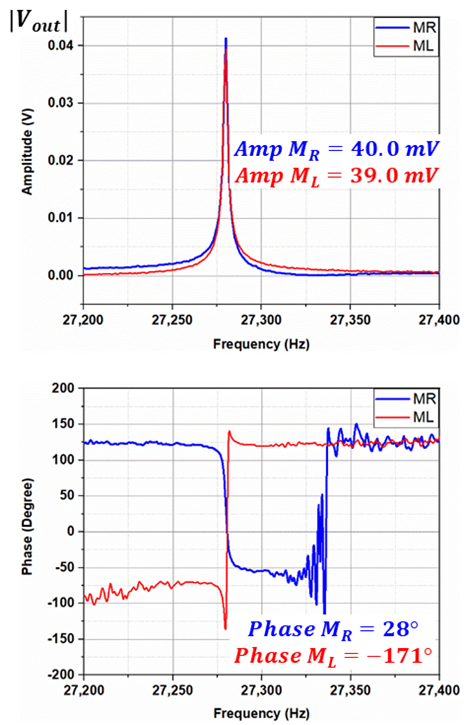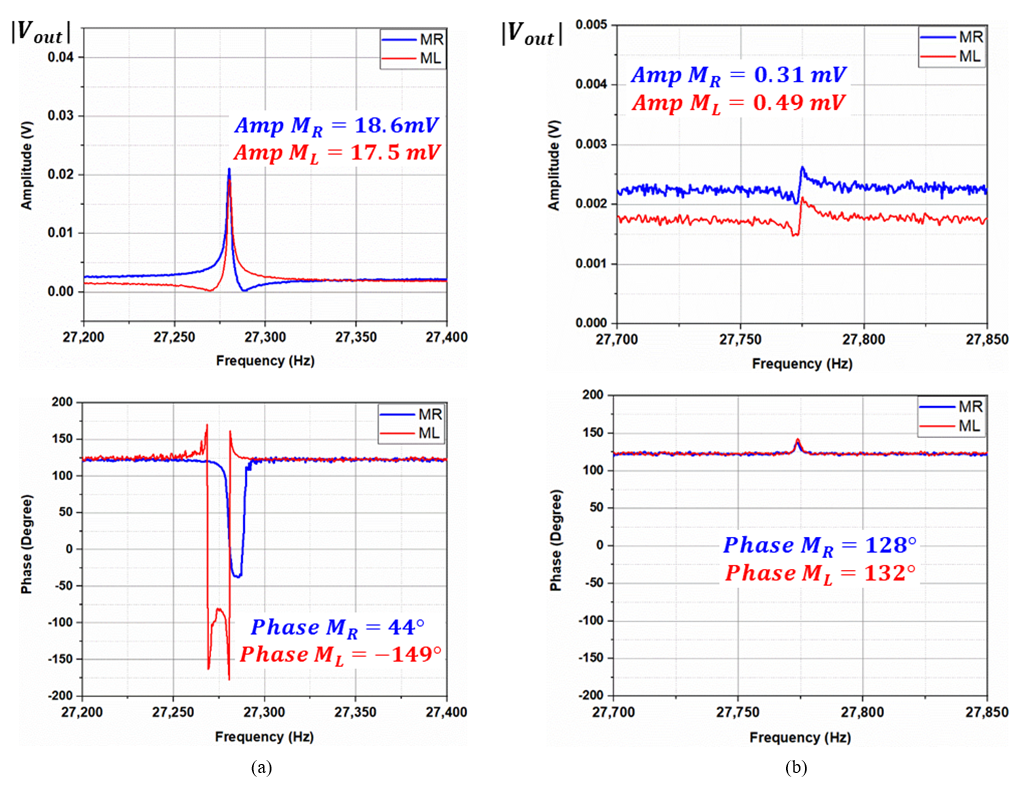Mobile QR Code1. (School of Mechatronics Engineering, Korea University of Technology and Education, 31253, Korea)
2. (Department of Robotics and Automation Engineering, Hoseo University, 31702, Korea)

MEMS, tuning fork, resonator

## I. INTRODUCTION

With improved performances, low cost, and compact size, MEMS tuning fork (MEMS-TF) structures are widely used as gyroscopes (1-3) and resonators (4-6). The MEMS-TF structures consist of two masses and are operated in anti-phase motion. These masses can be either coupled mechanically or electrostatically.

In electrostatically coupled resonators, it is challenging to achieve perfect anti-phase motion since the fabrication errors are inevitable, and it is almost impossible to fabricate two masses with the same frequency (7). To overcome this issue, mechanically coupled resonators have been proposed (8-10). One of the issues with mechanically coupled structures are the unwanted in-phase motion, which lies at a frequency lower than the required anti-phase frequency. Also, with the fabrication errors, both of the resonant modes can be merged.

MEMS-TF structures are believed to have the advantage of resisting linear accelerations, when operated in an anti-phase mode. However, structure asymmetry and spring unbalancing caused by the fabrication errors make MEMS-TF vulnerable to linear accelerations. The effect of acceleration sensitivity (g-sensitivity), on the performance of MEMS-TF structures has been presented widely (7,9,11-14), so it is essential to reduce the effect of linear accelerations (g-sensitivity) on these structures.

Several design approaches have been reported to improve the g-sensitivity of mechanically coupled MEMS tuning fork structures, Singh (10,15) studied the effect of linear accelerations, showing that the frequency decoupling ratio between the in-phase and anti-phase modes can improve the acceleration sensitivity. To further improve the resistance to linear accelerations and increase the decoupling ratios, different spring architectures are proposed, such as lever mechanism (16,17), z-beam coupling spring (18), diamond-shaped (19), circular-shaped (8,20), T-shaped (21), lozenge-shaped (22), and polygon-shaped (23). Most of the proposed spring architectures presented exhibit either large surface area or wide etch cavities. The large size of the spring will increase the device size, making it difficult to use in specific applications such as consumer electronics, whereas the wide etch area will make these springs more prone to fabrication errors.

In this article, a new coupling spring architecture has been proposed to overcome the previous spring designs' issues. The proposed design benefits with the miniaturization and narrow etch cavity, making it more robust to the fabrication errors. The proposed coupling spring always prioritizes the anti-phase motion by keeping the unwanted in-phase motion at a higher frequency, providing robustness to linear accelerations.

## II. THE DESIGN CONCEPT OF THE MEMS TUNING FORK RESONATOR

The MEMS tuning fork resonator's conceptual design, which can be modeled as two degrees of freedom system, is shown in Fig. 1. The two identical masses, left mass $(M_{L})~$and right mass$\left(M_{R}\right)$, are anchored through the left and right spring, $K_{L}$ and $K_{R},$respectively. Also, both the masses are mechanically coupled through coupling spring $K_{c}$ to form a shared resonance. The coupled resonators shown in Fig. 1 can be modeled as a second-order spring-mass system as shown below:

##### (1)
$$M_{L}\ddot{x}_{L}+C_{L}\dot{x}_{L}+K_{L}x_{L}+K_{c}\left(x_{L}- x_{R}\right)=F_{L}$$

##### (2)
$$M_{R}\ddot{x}_{R}+C_{R}\dot{x}_{R}+K_{R}x_{R}+K_{c}\left(x_{R}- x_{L}\right)=F_{R}$$

Here, $M,C,$ and $K~$represent the mass, damping, and spring constant of the resonator, and subscripts$R$and$L$represent the right and left sides.$F_{L}$ and $F_{R}$ are the applied forces. Eqs. (1) and (2) can be solved for the in-phase and anti-phase motions by assuming $M_{L}=M_{R}=M, C_{L}=C_{R}=C$ given by (6,19):

##### (3)
$$\ddot{x}_{in}+\frac{\omega _{in}}{Q_{in}}\dot{x}_{in}+\omega _{in}^{2}x_{in}=\frac{(F_{L}+F_{R})}{M}~ +\frac{K_{L}- K_{R}}{2M}x_{an}$$

##### (4)
$$\ddot{x}_{an}+\frac{\omega _{an}}{Q_{an}}\dot{x}_{an}+\omega _{an}^{2}x_{an}=\frac{(F_{L}- F_{R})}{M}~ - \frac{K_{R}- K_{L}}{2M}x_{in}$$

In this equation, $x_{an}=x_{L}- x_{R}$ is the anti-phase motion, $x_{in}=x_{L}+x_{R}$ is the in-phase motion, $\omega _{in}=$ $\sqrt{(K_{L}+K_{R})/M}$and$\omega _{an}=\sqrt{(K_{L}+K_{R}+4K_{c})/M}$represents the in-phase and anti-phase resonant frequencies, and $Q_{an}=M\omega _{an}/c$and$Q_{in}=M\omega _{in}/c$are the anti-phase and in-phase quality factors respectively.

From Eqs. (3) and (4), it is clear that the in-phase and anti-phase resonant modes depend on the polarity of the applied force and the coupling spring. The design goal for the coupling spring, as discussed above, is to have an anti-phase resonant frequency lower than the spurious in-phase resonant frequency, occupying a small surface area and narrow etch cavity. This can be achieved by the proposed anti-phase coupling spring architecture shown in the Fig. 2.

The proposed anti-phase spring architecture is comprised of outer, inner, and torsional arms. The outer arms are connected to the masses, $M_{L}$ and $M_{R}$. Meanwhile, one side of the inner arms is anchored, while the other side is connected to the outer arm through the torsional arm in a rotationally symmetric manner, constituting $180^{\circ}$ in relation to the center of the coupling spring. The deformation of the designed spring during the lateral load and axial load is shown in the Fig. 2(a) and (c) respectively. During the lateral load the outer arms move in the opposite direction, whereas the inner arms move in the same direction, in such a way that the torsional arm rotates along the lateral axis. The rotation of the torsional arm lessens the resistance for a low frequency anti-phase resonant mode. However, during the axial load, both the outer arms bend in the same direction, whereas the inner arms bend in the opposite direction. This opposite motion of the inner arm resist the motion of the torsional arm, resulting in a high frequency in-phase resonant mode.

The proposed spring architecture prioritizes the anti-phase motion, providing resistance to linear acceleration and robustness to fabrication imperfections with the narrow cavity holes. Resonant modes of the designed MEMS-TF resonator utilizing the anti-phase coupling spring architecture are shown in the Fig. 3.

## III. FEM SIMULATIONS

The designed MEMS-TF resonator was investigated through FEM simulation using COMSOL Multiphysics. The resonator was simulated as single-crystal silicon having a Young modulus of 179GPa and poison ratio of 0.29. A triangular mesh with a minimum element size of 0.1$\mathrm{\mu}m$ was used, consisting of 120 $\textit{K}$ triangular elements. Frequency domain study was used to investigate (i) the anti-phase operation of the proposed coupling spring and (ii) the effect of linear acceleration on the resonant modes of the MEMS-TF resonator.

### 3.1. Anti-phase Operation

FEM simulations validated the mechanically forced anti-phase motion. Initially, a balanced force of $0.2\mu N$ was applied to both the masses$M_{R}$ and $M_{L}$. The force $F_{R}$ and $F_{L}$ are acting on $M_{R}$ and $M_{L},$respectively, as shown in Fig. 4(a).

The frequency response function along with the phase is shown in Fig. 5(a). It can be observed that both masses are displaced in the anti-phase, having a maximum displacement of $3\mathrm{\mu}m$ at a resonant frequency of 29,760 $Hz$. This configuration is standard among MEMS-TF resonators to achieve anti-phase motion, also called electrical coupling or indirect coupling.

Fig. 5. The frequency response of the tuning fork structure (a) with balance actuation ($FL=-FR$), (b) frequency response of the tuning fork resonator when a single side force is applied.The frequency response of the tuning fork structure (a) with balance actuation ($FL=- FR$), (b) frequency response of the tuning fork resonator when a single side force is applied. The MEMS fabrication errors are inevitable, causing mass imbalance, spring imbalance or force imbalance to the tuning fork structure. To study the effect of imbalance force and robustness to the fabrication errors, one of the force was set to zero; i.e., $F_{R}=0$. This means that a single side force was applied to the MEMS-TF resonator. The frequency response function for this configuration is shown in Fig. 5(b). It can be observed that, the in-phase motion occurred at a higher frequency of 30,370 $Hz$. Here, it is worth mentioning that the anti-phase motion still dominants, having a displacement of $1.5\mathrm{\mu}m$.

### 2. Effect of Linear Accelerations

To study the effect of linear acceleration, a balanced force of $0.2\mu N$ was applied to both masses in opposite directions to actuate the MEMS-TF resonator differentially. Furthermore, a linear force was applied along the x-axis i.e. along the direction of actuation, as shown in Fig. 4(b). The linear force corresponds to a linear acceleration, ranging from $0G$ to $6G$, where, $1G=9.8m/sec^{2}$.

The effect of linear acceleration on the anti-phase resonant mode is shown in the Fig. 6(a). It can be observed that at $0G$ both the masses $M_{L}$ and $M_{R}$ had been displaced by $3\mathrm{\mu}m$ due to the balanced differential force, whereas the overall differential displacement of $6\mathrm{\mu}m$ was achieved. Furthermore, the displacement of both the independent masses, as well as the overall differential displacement was evaluated under the linear acceleration of $3G$ and $6G$. By linear fitting, it was observed that the linear acceleration sensitivity of the independent masses, $M_{L}$ and $M_{R}$ were$- 6nm/G$ and $5nm/G$ respectively, whereas the differential sensitivity was $1.1nm/G$.

Fig. 6. (a) Linear sensitivity of the anti-phase mode, (b) linear sensitivity of the in-phase mode.The same procedure was followed to study the effect of linear acceleration on the in-phase resonant mode, where the results are shown in the Fig. 6(b). It can be observed that at $0G,$ the net displacement i.e. both of the individual masses as well as differential displacement was zero, due to balance differential force. However, at high $~ G$, it was observed that the in-phase mode was more susceptible to the linear accelerations. The individual masses had an acceleration sensitivity of $89nm/G$, while the differential output corresponded to $76.4nm/G$. This shows that the anti-phase mode is $69.45$ times more resilient to the linear acceleration.

## IV. EXPERIMENTAL RESULTS

The equivalent electrical model and test method of the fabricated MEMS-TF resonator is shown in Fig. 7(a), while the optical image of the fabricated device using the designed coupling spring is shown in Fig. 7(b). The resonator was fabricated using three wafer stacked processes (i.e., device wafer, via wafer, and cap wafer), with a device thickness of $30\mu m$ and vacuum-sealed at $100\textit{mtorr}$.

Fig. 7. (a) Electrical modal and experimental setup, (b) optical photograph of wafer-level vacuum packaged MEMS-TF resonator (captured by EMI PHEMOS-1000).The resonator was actuated by electrostatic force with an $AC~$ voltage of$v_{ac}=100mV_{pk}$ and a $DC$ polarization voltage of $V_{DC}=5V$. The drive circuitry generates the differential signal; i.e., $V_{DP}=V_{DC}+v_{ac}$ and $V_{DN}=V_{DC}- v_{ac}$. The sense current was converted to the sense voltage using a charge amplifier, and the frequency response function (FRF) was computed through the Zurich instrument (MFLI).

### 4.1.1. Differential Balance Force

The frequency response of the left mass and right mass with balance differential force is depicted in Fig. 8, showing both the masses moving in anti-phase motion (i.e., 180˚ out of phase, with an amplitude response of $40mV$ and $39mV$). The anti-phase resonant frequency was $27,280Hz$. A comparison of simulation and experimental results depicted a phase error of $19^{o}$ between the two masses. It was found that the phase error arises from the parasitic feed-through signal, i.e. the drive signal has been coupled to sense signal through parasitic capacitances. The phase error can be minimized by implementing the feed-through cancellation scheme as discussed in (2).

Fig. 8. Anti-phase resonant mode with balance-anti-phase force.#### 4.1.2. Single Side Force

When actuated by the single side, the resonant masses' frequency response is shown in Fig. 9. During the anti-phase motion, as shown in Fig. 9(a), it can be observed that the output voltage is almost halved of the amplitude, $18mV$, compared to the differential actuation, since the force is halved, while the phase error was measured to be $13^{\circ}$. Meanwhile, the in-phase motion was observed at a higher frequency of $27,780Hz$, with an amplitude of $0.4mV$, as shown in Fig. 9(b). It is worth mentioning that, with the single side force, the in-phase mode is suppressed by $97.7\,\,\mathrm{\% }$, while the coupling spring prioritizes the anti-phase motion.

Fig. 9. Frequency response of the MEMS-TF resonator with single side force (a) anti-phase response, (b) in-phase response.## V. CONCLUSION

This study has successfully demonstrated a new coupling spring design for MEMS tuning fork structures. The new coupling spring design provides perfect anti-phase motion at a lower resonant mode while keeping the surplus in-phase resonant mode high. The designed coupling spring has low etched cavities, making it more robust to fabrication errors and small surface area, resulting in a wide range of device applications. The FEM simulations were carried out to study the effect of linear accelerations and un-balanced forces. The in-phase mode is more susceptible to the linear accelerations, showing that the individual masses have an acceleration sensitivity of $89nm/G$, whereas the differential output corresponds to $76.4nm/G$. This shows that the anti-phase mode is 69.45 times more resilient to the linear acceleration. Furthermore, experimentally, the designed coupling spring architecture suppresses the in-phase mode by 97 %.

Author Contributions: Conceptualization, Faisal Iqbal, Seungoh Han and Byeungleul Lee; Formal analysis, Faisal Iqbal; Software, Faisal Iqbal and Hussamud Din; Experiment, Faisal Iqbal; Supervision, Byeungleul Lee; Writing—original draft, Faisal Iqbal; Writing—review and editing, Faisal Iqbal, Hussamud Din, and Byeungleul Lee.

### ACKNOWLEDGMENTS

This work is supported by the R&D program of the Ministry of Trade, Industry, and Energy (MOTIE)/Korea Evaluation Institute of Industrial Technology (KEIT). (10084665, Development of IMU Embedded 6-axis, 10-axis compound navigation system integrating highly reliable inertial measurement unit (IMU), Global Navigation Satellite System (GNSS), Magnetometer, and altimeter for maned/unmanned aircraft). This work was (partially) supported by the post-doc scholarship program of KOREATECH.

### REFERENCES

1
Iqbal F., Din H., Lee B., 2019, Single Drive Multi-Axis Gyroscope with High Dynamic Range, High Linearity and Wide Bandwidth, Micromachines, Vol. 102
Iqbal F., Shah M. A., Lee B., 2017, Analysis of parasitic feed-through capacitance in MEMS gyroscope with push pull configuration, IEEE 12th International Conference on Nano/Micro Engineered and Molecular Systems (NEMS), pp. 414-4173
Walther A., Le Blanc C., Delorme N., Deimerly Y., Anciant R., Willemin J., 2012, Bias contributions in a MEMS tuning fork gyroscope, Journal of microelectromechanical systems, Vol. 22, No. 22, pp. 303-3084
Iqbal F., Lee B., 2018, A Study on Measurement Variations in Resonant Characteristics of Electrostatically Actuated MEMS Resonators, Micromachines, p. 173, Vol. 95
Roessig T.-A. W., 1999, Integrated MEMS tuning fork oscillators for sensor applications, 1999.6
Zotov S. A., Simon B. R., Prikhodko I. P., Trusov A. A., Shkel A. M., 2014, Quality factor maximization through dynamic balancing of tuning fork resonator, IEEE Sensors Journal, Vol. 14, pp. 2706-27147
Janioud P., Koumela A., Poulain C., Rey P., Berthelot A., Morfouli P., 2017, Tuning the Anti-Phase Mode Sensitivity to Vibrations of a MEMS Gyroscope, in Multidisciplinary Digital Publishing Institute Proceedings, p. 3558
Azgin K., Temiz Y., Akin T., 2007, An SOI-MEMS tuning fork gyroscope with linearly coupled drive mechanism, IEEE 20th International Conference on Micro Electro Mechanical Systems (MEMS), Vol. , No. , pp. 607-6109
Gao Y., Li H., Huang L., Sun H., 2017, A lever coupling mechanism in dual-mass micro-gyroscopes for improving the shock resistance along the driving direction, Sensors, p. 995, Vol. 1710
Thakur P. S., Sugano K., Tsuchiya T., Tabata O., 2014, Experimental verification of frequency decoupling effect on acceleration sensitivity in tuning fork gyroscopes using in-plane coupled resonators, Microsystem technologies, Vol. 20, pp. 403-41111
Yoon S. W., Lee S., Najafi K., 2012, Vibration-induced errors in MEMS tuning fork gyroscopes, Sensors and Actuators A: Physical, Vol. 180, pp. 32-4412
Koumela A., Poulain C., Le Goc C., Verdot T., Joet L., Rey P., 2016, Resilience to vibration of a tuning fork MEMS gyroscope, Procedia Engineering, Vol. 168, pp. 1725-173013
Guan Y., Gao S., Liu H., Niu; S., 2015, Acceleration sensitivity of tuning fork gyroscopes: Theoretical model, simulation and experimental verification, Microsystem Technologies, Vol. 21, pp. 1313-132314
Giacci F., Dellea S., Langfelder; G., 2017, Signal integrity in capacitive and piezoresistive single-and multi-axis MEMS gyroscopes under vibrations, Microelectronics Reliability, Vol. 75, pp. 59-6815
Singh T. P., Sugano K., Tsuchiya T., Tabata; O., 2012, Frequency response of in-plane coupled resonators for investigating the acceleration sensitivity of MEMS tuning fork gyroscopes, Microsystem technologies, Vol. 18, pp. 797-80316
Trusov A., Schofield A., Shkel A., 2009, Gyroscope architecture with structurally forced anti-phase drive-mode and linearly coupled anti-phase sense-mode, in TRANSDUCERS 2009-2009 Inter-national Solid-State Sensors, Actuators and Microsystems Conference, pp. 660-66317
Trusov A. A., Schofield A. R., Shkel; A. M., 2011, Micromachined rate gyroscope architecture with ultra-high quality factor and improved mode ordering, Sensors and Actuators A: Physical, Vol. 165, pp. 26-3418
Din H., Iqbal F., Lee; B., 2020, Modelling and optimization of single drive 3-axis MEMS gyroscope, Microsystem Technologies, pp. 1-919
Guan Y., Gao S., Liu H., Jin L., Niu; S., 2016, Design and vibration sensitivity analysis of a MEMS tuning fork gyroscope with an anchored diamond coupling mechanism, Sensors, p. 468, Vol. 1620
Guan Y., Gao S., Jin L., Cao; L., 2016, Design and vibration sensitivity of a MEMS tuning fork gyroscope with anchored coupling mechanism, Microsystem Technologies, Vol. 22, pp. 247-25421
Sharma A., Zaman F. M., Amini B. V., Ayazi; F., 2004, A high-Q in-plane SOI tuning fork gyroscope, in SENSORS, IEEE, Vol. 2004 ieee, pp. 467-47022
Trinh T. Q., Nguyen L. Q., Dao D. V., Chu H. M., Vu; H. N., 2014, Design and analysis of a z-axis tuning fork gyroscope with guided-mechanical coupling, Microsystem technologies, Vol. 20, pp. 281-28923
Xu Q., Hou Z., Kuang Y., Miao T., Ou F., Zhuo M., 2019, A tuning fork gyroscope with a polygon-shaped vibration beam, Micromachines, p. 813, Vol. 10## Author

Faisal Iqbal received his B.S. degree in Telecommunication Engi-neering from University of Engineering and Technology, Peshawar, Pakistan.

He received his MS and Ph.D. degree in Interdisciplinary Program in Creative Engineering from Korea University of Technology and Education, Republic of Korea, in 2017 and 2020, respectively.

He is currently working as a Research Fellow at Queen’s University Belfast, United Kingdom.

Previously, he worked as Postdoctoral researcher at Korea University of Technology and Education for 1 year. His research interests include MEMS based inertial sensors.

Hussamud din received his B.S. degree in Electronics Engineering from International Islamic University, Islamabad, Pakistan in 2011, and M.S. degree in Electrical Engi-neering from Center for Advanced Studies in Engineering, Islamabad, Pakistan in 2016.

He is currently pursuing his Ph.D. degree in Mechatronics Engineering at Korea University of Technology and Education, Cheonan, South Korea.

His research interests include MEMS inertial sensors and energy harvesters.

Seungoh Han received his B.S., M.S., and Ph.D. degrees in electrical engineering at Korea University (Seoul, Korea) in 1996, 1998, and 2006, respectively.

Since 2007, he has been working for Hoseo University (Asan, Korea) as a professor.

His current research interests include smart sensors, MEMS/NEMS, and coupled-physics analysis of micro/nano-devices.

Byeungleul Lee is Professor at Korea University of Technology and Education.

He received his B.S. degree in Electronics Engineering from the Hanyang University, and M.S. degree in Electrical and Electronics engineering from Korea Advanced Institute of Technology in 1989 and 1991, respectively.

He obtained his Ph.D. in Electrical Engineering and Computer Science from the Seoul National University in 2004.

From 1991 to 2008, he worked for Samsung Electronics as a principal researcher for MEMS development. His research interests include semiconductor transducer and MEMS applications.